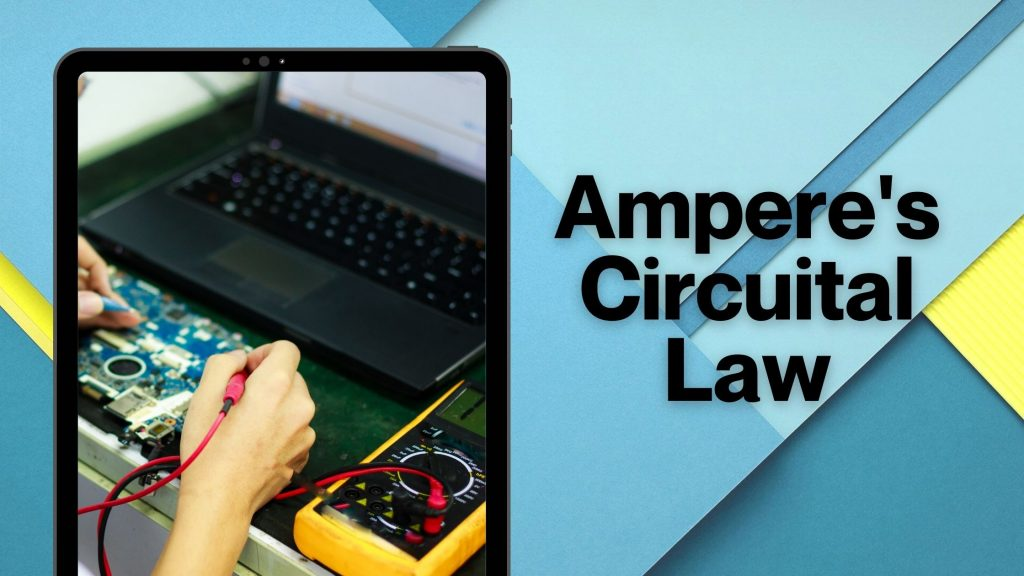Student
Study Material

# Ampere’s Law | Amperes Circuital Law

Ampere's circuital law states that the line integral of magnetic field around a closed path is equal to the product of the permeability and current.

5 minutes long
Posted by Mahak Jain, 3/11/2021Hesap Oluştur

Got stuck on homework? Get your step-by-step solutions from real tutors in minutes! 24/7. Unlimited.

The ampere’s law defines the relationship between the magnetic field and current that it generated around itself due to current or vice versa. Ampere’s Circuital Law is its application in circuit. This is explained in detail below.

## What is Ampere’s Law

According to Ampere’s law, magnetic fields are related to the electric current produced in them. The ampere’s circuital law is different in a way that it is for a closed loop.

Consider an open surface with a boundary. The surface is like that electric current passing through it.

Now we can consider that the boundary is made up of a number of small line elements. Suppose Length of one such element is dl. The value of the tangential component of the magnetic field, B at this element.

Now multiply magnetic field B by the length of that element dl. Add all such products together. The sum then tends to an integral. According to ampere’s law this integral is equal to µ0 times the total current passing through the surface.

As ∫B.dI = µ₀I

Thus The law specifies the magnetic field that is associated with a given current or vice-versa.

## State Ampere’s Circuital Law

Ampere’s circuital law states that,’ the line integral of magnetic field around a closed path is equal to the product of the magnetic permeability of that space and the total current through the area bounded by that path”.

∮B.dl=μ₀I

∮B.dl = line integral of B around a closed path.

Here B = magnetic field which is a magnetic field on moving electric charge, magnetized materials, and electric currents.

μ₀ = Magnetic permeability of free space

μ₀ = 4 π × 10-7 N/A2

## Derivation of Ampere’s Circuital Law

Consider a small coil carrying current l.

Let assume this coil is made up of numerous small elements dl. Now consider this small element dl on loop. Then

∮B.dl =  ∮B dl cos θ

Here θ is angle with the magnetic field

The magnetic field will be around the conductor so

θ = 0°

In a long wire carrying current, the magnetic field at point P at a perpendicular distance ‘r’ from the conductor is given as

B =  µ₀I / 2πr

Wire is in coil format. So, ∫dl = 2πr

∮B.dl = µ₀I/2πr × 2πr

Thus, ∮B.dl = µ₀I

## Ampere’s Law Equation

The line Integral of the magnetic field is equal to the product of magnetic permeability µ₀ and current l flowing through it.

∮B.dl = µ₀I

Here B is Magnitude of magnetic field

∮B.dl is the line Integral of the magnetic field

µ₀ is magnetic permeability

I is the current flowing through coil.

## Applications of Ampere’s Circuital Law

### Magnetic Field Due to Long Straight Wire

The current I flowing through straight wire as

magnetic field at perpendicular distance ‘a’ at point P,

If wire is long wire or infinite length wire then In this condition point P always be at centre of straight wire so the angle ϕ1 & ϕ2 will be equal to 90°

Now Sin ϕ1 = Sin ϕ2 = Sin 90° = 1

B = µ₀I (1 + 1) / 4πa

Thus, B = µ₀I / 2πa

Therefore, magnetic field due to long straight wire at distance a is B = µ₀I / 2πa

Magnetic field B is directly proportional to current I and inversely proportional to perpendicular distance ‘a’.

### Calculation of Magnetic Field Due to Solenoid

It consists of a long wire wound in the form of a helix where the subsequent turns present very closely . Each turn is such closely spaced that each turn can be regarded as a circular loop. Therefore net magnetic field for whole solenoids will be the vector sum of the fields due to all the individual turns.

Magnetic field due to solenoids B = µ°nI

The magnetic field due to a section of the solenoid

Solenoids are uses in television to generate magnetic field.

### Magnetic Field Due to Toroid

The toroid is a hollow circular ring on which a large number of turns of a wire are closely wound.

A sectional view of the toroid,

Magnetic field due to toroid B = µ₀NI / 2πr.